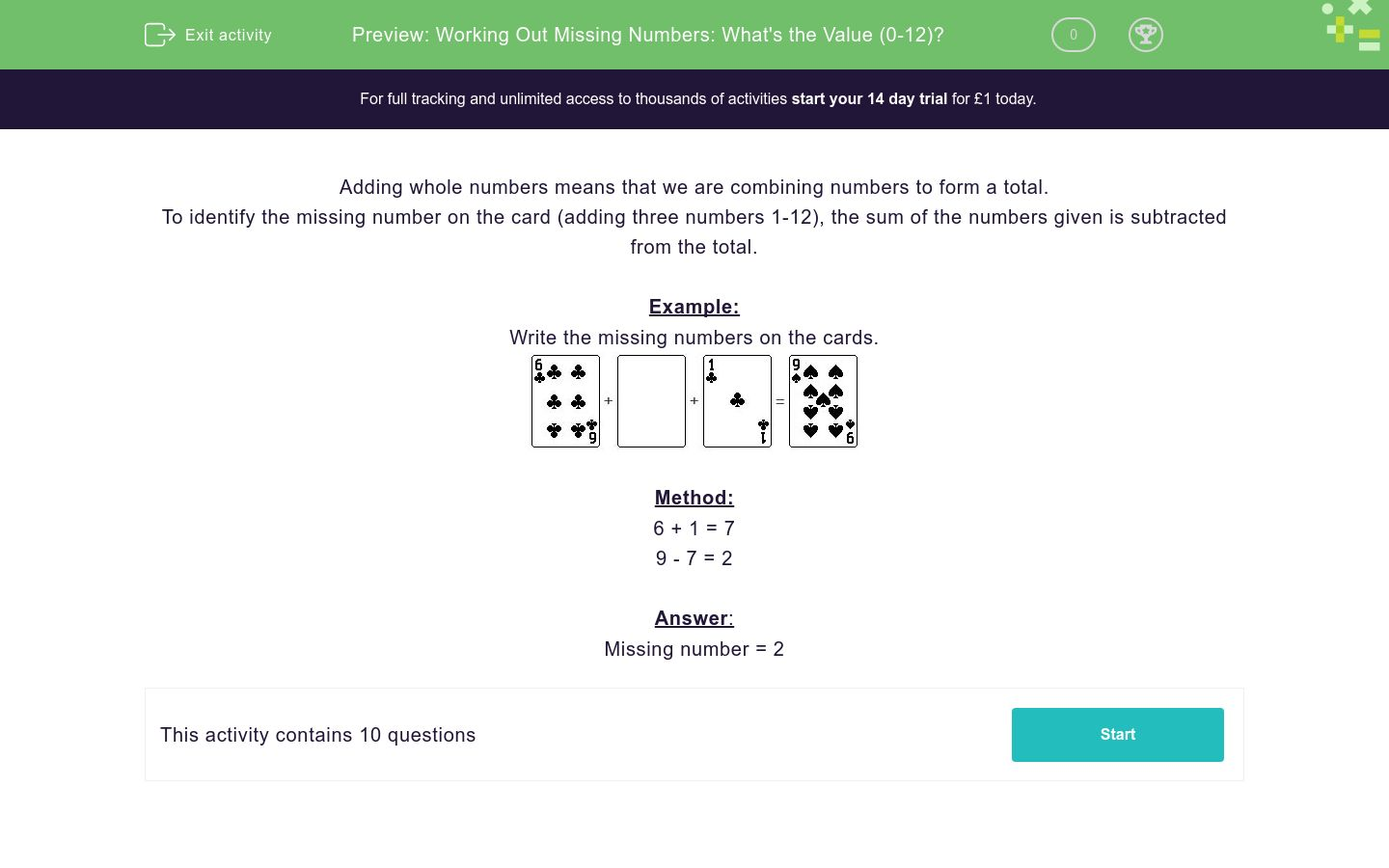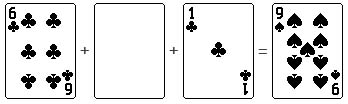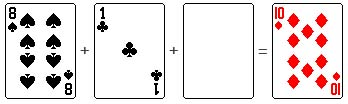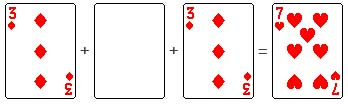# Working Out Missing Numbers: What's the Value (0-12)?

In this worksheets, students identify the missing numbers on the cards (adding three numbers 0-12).Key stage:  KS 1

Curriculum topic:   Number: Addition and Subtraction

Curriculum subtopic:   Solve Add/Subtract Problems to 100

Difficulty level:### QUESTION 1 of 10

Adding whole numbers means that we are combining numbers to form a total.

To identify the missing number on the card (adding three numbers 1-12), the sum of the numbers given is subtracted from the total.

Example:

Write the missing numbers on the cards.Method:

6 + 1 = 7

9 - 7 = 2

Missing number = 2

Write the missing numbers on the card.Write the missing numbers on the card.Write the missing numbers on the card.Write the missing numbers on the card.Write the missing numbers on the card.Write the missing numbers on the card.Write the missing numbers on the card.Write the missing numbers on the card.Write the missing numbers on the card.Write the missing numbers on the card.• Question 1

Write the missing numbers on the card.2
EDDIE SAYS

Method

4 + 2 = 6

8 - 6 = 2

Missing Number = 2

• Question 2

Write the missing numbers on the card.1
EDDIE SAYS

Method

4 + 3 = 7

8 - 7 = 1

Missing Number = 1

• Question 3

Write the missing numbers on the card.1
EDDIE SAYS

Method

8 + 1 = 9

10 - 9 = 1

Missing Number = 1

• Question 4

Write the missing numbers on the card.8
EDDIE SAYS

Method

1 + 1 = 2

10 - 2 = 8

Missing Number = 8

• Question 5

Write the missing numbers on the card.1
EDDIE SAYS

Method

6 + 2 = 8

9 - 8 = 1

Missing Number = 1

• Question 6

Write the missing numbers on the card.5
EDDIE SAYS

Method

3 + 1 = 4

9 - 4 = 5

Missing Number = 5

• Question 7

Write the missing numbers on the card.2
EDDIE SAYS

Method

4 + 2 = 6

8 - 6 = 2

Missing Number = 2

• Question 8

Write the missing numbers on the card.2
EDDIE SAYS

Method

6 + 1 = 7

9 - 7 = 2

Missing Number = 2

• Question 9

Write the missing numbers on the card.1
EDDIE SAYS

Method

3 + 3 = 6

7 - 6 = 1

Missing Number = 1

• Question 10

Write the missing numbers on the card.5
EDDIE SAYS

Method

2 + 1 = 3

8 - 3 = 5

Missing Number = 5

---- OR ----

Sign up for a £1 trial so you can track and measure your child's progress on this activity.

### What is EdPlace?

We're your National Curriculum aligned online education content provider helping each child succeed in English, maths and science from year 1 to GCSE. With an EdPlace account you’ll be able to track and measure progress, helping each child achieve their best. We build confidence and attainment by personalising each child’s learning at a level that suits them.

Get started